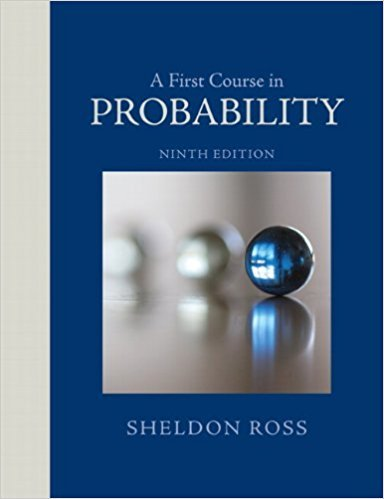×
Get Full Access to A First Course In Probability - 9 Edition - Chapter 3 - Problem 13ste
Get Full Access to A First Course In Probability - 9 Edition - Chapter 3 - Problem 13ste

×

# Balls are randomly removed from an urn that initiallyISBN: 9780321794772 63

## Solution for problem 13STE Chapter 3

A First Course in Probability | 9th Edition

• Textbook Solutions
• 2901 Step-by-step solutions solved by professors and subject experts
• Get 24/7 help from StudySoup virtual teaching assistantsA First Course in Probability | 9th Edition

4 5 1 378 Reviews
16
1
Problem 13STE

Problem 13STE

Balls are randomly removed from an urn that initially contains 20 red and 10 blue balls.

(a) What is the probability that all of the red balls are removed before all of the blue ones have been removed? Now suppose that the urn initially contains 20 red, 10 blue, and 8 green balls.

(b) Now what is the probability that all of the red balls are removed before all of the blue ones have been removed?

(c) What is the probability that the colors arc depleted in the order blue, red, green?

(d) What is the probability that the group of blue balls is the first of the three groups to be removed?

Step-by-Step Solution:

Solution:

Step 1 of 4:

1. An urn contains 20 red and 10 blue balls and the balls are removed randomly.

The claim is to find the probability that all of the red balls are removed before all of the blues have been removed.

Then P(last removed ball is blue) === 0.3333

Hence, the probability that all of the red balls are removed before all of the blues has been removed is 0.3333.

Step 2 of 4

Step 3 of 4

##### ISBN: 9780321794772

This textbook survival guide was created for the textbook: A First Course in Probability , edition: 9. The full step-by-step solution to problem: 13STE from chapter: 3 was answered by , our top Statistics solution expert on 08/11/17, 08:57AM. This full solution covers the following key subjects: balls, removed, Blue, red, Probability. This expansive textbook survival guide covers 4 chapters, and 469 solutions. The answer to “Balls are randomly removed from an urn that initially contains 20 red and 10 blue balls.(a) What is the probability that all of the red balls are removed before all of the blue ones have been removed? Now suppose that the urn initially contains 20 red, 10 blue, and 8 green balls.________________(b) Now what is the probability that all of the red balls are removed before all of the blue ones have been removed?________________(c) What is the probability that the colors arc depleted in the order blue, red, green?________________(d) What is the probability that the group of blue balls is the first of the three groups to be removed?” is broken down into a number of easy to follow steps, and 109 words. A First Course in Probability was written by and is associated to the ISBN: 9780321794772. Since the solution to 13STE from 3 chapter was answered, more than 984 students have viewed the full step-by-step answer.

Unlock Textbook Solution